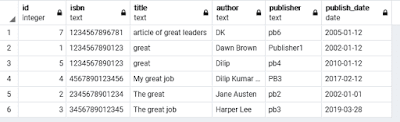### SQL Server - How to find all empty/have values tables

Many times our managers ask to find empty tables and have value tables report. It is time taken to find the tables manually. I am writing some queries which may help you to find empty and have a values table.
The following query helps you to find all empty tables in the database.

1 – See the following query

 SELECT IST.TABLE_NAME , MAX(SI.rows ) TOTAL_RECORDS FROM sysindexes SI, INFORMATION_SCHEMA.TABLES IST WHERE IST.TABLE_NAME = OBJECT_NAME(SI.id)       AND IST.TABLE_TYPE = 'BASE TABLE' GROUP BY IST.TABLE_SCHEMA, IST.TABLE_NAME HAVING MAX(SI.rows)<=0

2- If you want to find all empty tables of particular “schema” then use the following query.

 SELECT IST.TABLE_NAME , MAX(SI.rows ) TOTAL_RECORDS FROM sysindexes SI, INFORMATION_SCHEMA.TABLES IST WHERE IST.TABLE_NAME = OBJECT_NAME(SI.id)       AND IST.TABLE_TYPE = 'BASE TABLE'       AND IST.TABLE_SCHEMA='Schema_Name' GROUP BY IST.TABLE_SCHEMA, IST.TABLE_NAME HAVING MAX(SI.rows)<=0

If you want to find those all tables that have values, then use the following queries.
1 – Query to find all tables that have values.

 SELECT IST.TABLE_NAME , MAX(SI.rows ) TOTAL_RECORDS FROM sysindexes SI, INFORMATION_SCHEMA.TABLES IST WHERE IST.TABLE_NAME = OBJECT_NAME(SI.id)       AND IST.TABLE_TYPE = 'BASE TABLE' GROUP BY IST.TABLE_SCHEMA, IST.TABLE_NAME HAVING MAX(SI.rows)>0

2- If you want to find all tables that have values of particular “schema,” then use the following query.

 SELECT IST.TABLE_NAME , MAX(SI.rows ) TOTAL_RECORDS FROM sysindexes SI, INFORMATION_SCHEMA.TABLES IST WHERE IST.TABLE_NAME = OBJECT_NAME(SI.id)       AND IST.TABLE_TYPE = 'BASE TABLE'       AND IST.TABLE_SCHEMA = 'SCHEMA_NAME' GROUP BY IST.TABLE_SCHEMA, IST.TABLE_NAME HAVING MAX(SI.rows)>0

 ;WITH TBL AS (    SELECT       SUM(row_count) AS ROW_COUNT,       OBJECT_NAME(OBJECT_ID) AS TABLE_NAME,       OBJECT_SCHEMA_NAME(OBJECT_ID) AS TABLE_SCHEMA    FROM       sys.dm_db_partition_stats    WHERE       INDEX_ID = 0 OR INDEX_ID= 1              GROUP BY       OBJECT_ID ) SELECT * FROM TBL WHERE ROW_COUNT = 0

You can filter for schema as below.

 ;WITH TBL AS (    SELECT       SUM(row_count) AS ROW_COUNT,       OBJECT_NAME(OBJECT_ID) AS TABLE_NAME,       OBJECT_SCHEMA_NAME(OBJECT_ID) AS TABLE_SCHEMA    FROM       sys.dm_db_partition_stats    WHERE       INDEX_ID = 0 OR INDEX_ID= 1              GROUP BY       OBJECT_ID ) SELECT * FROM TBL WHERE ROW_COUNT = 0 AND TABLE_SCHEMA='Schema_Name'

If you wish to find all tables which have values so please change in above query ROW_COUNT >0

### PostgreSQL-Query: Sort result set by specific field values using ORDER BY Clause

Problem: Suppose we have a book_inventory table which has some columns such as id, isbn, title, author, publisher, publish_date, etc.. whe...